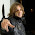## Jun 4, 2011

### Math.Round Utility in Salesforce

Now rounding your number to desired decimal value is no longer a headache.

How?????

Just use the below method and pass your decimal number as first parameter and the decimal places as second parameter.
```public Decimal roundNumber(Decimal roundNumber , Integer decimalPlace)
{
if(roundNumber != null)
{
Decimal decPlace = Math.POW(10 , decimalPlace) ;
return Math.round(roundNumber * decPlace) / decPlace ;
}
else
{
return 0 ;
}
}
```

Presently salesforce provides Math.Round(your_number) which will return the number after rounding with no decimal values.

For example : If I do this
```System.debug('Rounded number : ' + Math.Round(2.333454)) ;
```

It will return Round Number : 2

I don't find way to get number round with two decimal places like in this case if I want the number to be rounded with two decimal places then it should return 2.33

Above method makes it pretty simple, we only need to pass decimal value and the decimal places in method like this :
```Decimal num = roundNumber(1.567 , 2) ;
System.debug('num ::::: ' + num) ;
```
It will return 1.57

Also another way to round number by decimal places is :
```Decimal d = 1.426 ;
Double num = d.setScale(2) ;
System.debug('num ::::::::::::: ' + num) ;
```

Cheers
Feedbacks are always welcomed

1.This comment has been removed by the author.

2.Decimal num = roundNumber(1.567 , 2) ;
System.debug('num ::::: ' + num) ;

It will return 1.57.
--------------------------------------------------
I believe you have made mistake . I think it must be 1.56 )

3.Have you tried this??
I think this is right as I want numbre to be rounded with 2 decimal places and if I am rounding the number 1.567 then it should return 1.57 as 7 is greater than 4. If I have written 1.563 then it should return 1.56 as 3 is smaller than 4.

1.Hi ankit,

Please help in calculatine me Amortization Amount. Following is the detail and Formula. and please let me know how can we do in Apex.

r = Interest/period 1%
n = Number of period 36
P = Principal amount \$10,000.00
A = Amortization

Formula: =+E9*((E7*(1+E7)^E8)/((1+E7)^E8-1))

There is one PMT method in Excel that will give result \$332.14 for above values.

This is the PMT function in Excel
=-PMT(E7,E8,E9)

4.This function is cool. However it wasn't correct when I tried to round a large number

roundNumber(50000000.24567, 2) -> 7050327.29
roundNumber(22000000.24567, 2) -> -20949672.71

5.Decimal num = roundNumber(1.567 , 2) ;
System.debug('num ::::: ' + num) ;

This is giving error.
Method does not exist or incorrect signature: roundNumber(Decimal, Integer).

6.Cool it works! ,,,, Also can we round the size of the Input File (uploaded from VF page) which is sent to amazonS3 from force.com... which has file properties such as
public Blob fileBlob {get;set;}
public Decimal fileSize {get;set;} // which takes size of the file; Can i round this value to nearest megabyte value and show the same both in vf page and S3 for the file uploaded
public String fileName {get;set;}

Help Me.

7.Decimal cutPrice = ((invoice.Price__c / 100) * info.Assessment__r.Discount__c);
System.debug(finalPrice);

System.debug(cutPrice.setScale(2));

8.Hi ankit,

Please help in calculatine me Amortization Amount. Following is the detail and Formula. and please let me know how can we do in Apex.

r = Interest/period 1%
n = Number of period 36
P = Principal amount \$10,000.00
A = Amortization

Formula: =+E9*((E7*(1+E7)^E8)/((1+E7)^E8-1))

There is one PMT method in Excel that will give result \$332.14 for above values.

9.This is the PMT function in Excel
=-PMT(E7,E8,E9)

10.I want to validate postal code using controller validation how do I do it?
I'm new to salesforce.
postal code: data type(Number(4,0))

11.I am really enjoying to read your article. Thank you for sharing this wonderful information. As, you can also take advantages to get the Impact Doors Services in Miami. These service need to secure your life's.